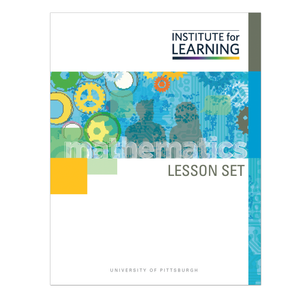# Solving One-Variable Equations and Inequalities (Grade 6)

Regular price \$6.25 \$0.00 Unit price per

This lesson set provides an introduction to one-variable equations and inequalities. The set starts by working on writing and solving one-variable equations. The last few tasks expose students to transitioning from equations to inequalities.

Through engaging in the lessons in this lesson set, students will:

• translate contexts into one-variable equations and inequalities;
• determine the meaning of the variable and numbers in an equation as they relate to a context in order to solve problems with equations; and
• use inverse operations to isolate a variable; or if the variable solution is known, will substitute and evaluate.

Standards: 6.EE.B.5, 6.EE.B.6, 6.EE.B.7, 6.EE.B.8